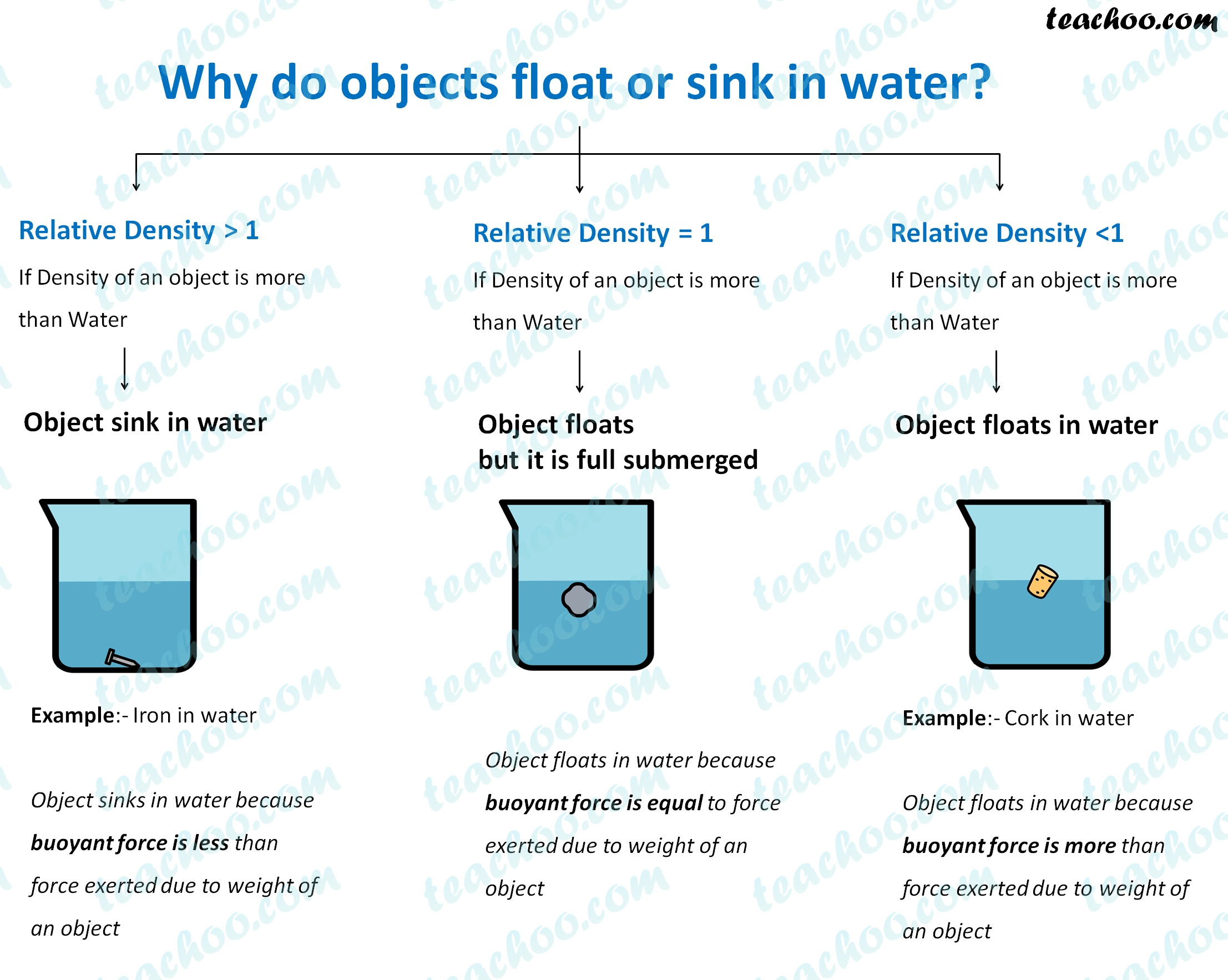Concepts

Class 9
Chapter 10 Class 9 - Gravitation

## Why do some Objects sink in Water

Those objects which have density more than water sink in water

We can also say that those having Relative Density more than 1 sink in water

Example

Iron floats in water because Iron has more density than water

(Density of iron is 7800 kg/m 3 but water is 1000 kg/m 3

Relative Density of Iron = Density of iron/Density of Water = 7800/1000 = 7.8

We can also say that since relative density of object is more than 1, it sinks in water

## Reason for Sinking

The object sinks in water because buoyant force is less than force exerted due to weight of object due to its high density

Why do Some Objects float in water

Those objects which have density less than water float in water

We can also say that those having Relative Density less than 1 sink in water

Example

Cork floats in water because cork has less density than water

(Density of Cork is 240 kg/m 3 but water is 1000 kg/m 3

Relative Density of Cork = Density of Cork/Density of Water = 240/1000 = 0.24

We can also say that since relative density of object is less than 1, it floats in water

## Reason for Floating

The object floats in water because buoyant force is more than force exerted due to weight of object due to its low density## Ships are made of iron built still it floats in Water. Why?

Ship is made of iron and steel

But is a hollow object containing lot of air

Air has lower density than water

So upward buoyant force of water is high which keeps ship afloat

## Questions

NCERT Question 21 - The volume of 50 g of a substance is 20 cm 3 . If the density of water is 1 g cm –3 , will the substance float or sink?

Learn in your speed, with individual attention - Teachoo Maths 1-on-1 Class

### Transcript

Why do objects float or sink in water? Relative Density > 1 If Density of an object is more than Water Relative Density = 1 If Density of an object is more than Water Relative Density <1 If Density of an object is more than Water Object sink in water Object floats but it is full submerged Object floats in water Example:- Iron in water Object sinks in water because buoyant force is less than force exerted due to weight of an object Object floats in water because buoyant force is equal to force exerted due to weight of an object Example:- Cork in water Object floats in water because buoyant force is more than force exerted due to weight of an object